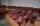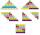Cans

How many cans must be put in the bottom row if we want 182 cans arrange in 13 rows above so that each subsequent row has always been one tin less? How many cans will be in the top row?

Result

a =  20
b =  8

Solution:Leave us a comment of example and its solution (i.e. if it is still somewhat unclear...):Be the first to comment!To solve this verbal math problem are needed these knowledge from mathematics:

Do you have a linear equation or system of equations and looking for its solution? Or do you have quadratic equation?

Next similar examples:

1. SeatsSeats in the sport hall are organized so that each subsequent row has five more seats. First has 10 seats. How many seats are: a) in the eighth row b) in the eighteenth row
2. Theorem proveWe want to prove the sentence: If the natural number n is divisible by six, then n is divisible by three. From what assumption we started?
3. SequenceBetween numbers 1 and 53 insert n members of the arithmetic sequence that its sum is 702.
4. SequenceWrite the first 6 members of these sequence: a1 = 5 a2 = 7 an+2 = an+1 +2 an
5. AS sequenceIn an arithmetic sequence is given the difference d = -3 and a71 = 455. a) Determine the value of a62 b) Determine the sum of 71 members.
6. AP - simpleDetermine the first nine elements of sequence if a10 = -1 and d = 4
7. Sequence 3Write the first 5 members of an arithmetic sequence: a4=-35, a11=-105.
8. Sequence 2Write the first 5 members of an arithmetic sequence a11=-14, d=-1
9. SequenceWrite the first 7 members of an arithmetic sequence: a1=-3, d=6.
10. ChairsDetermine the number of seats in the seventh row and ninth row, if 3rd row has 14 seats and in every next row of seats has five more than the previous row.
11. Consecutive numbersSum of ten consecutive numbers is 105. Determine these numbers (write first and last).
12. AP 6Calculate the first five items of an arithmetic sequence if it is given: a2 – a3 + a5 = 20 a1 + a6 = 38
13. Fifth memberDetermine the fifth member of the arithmetic progression, if the sum of the second and fifth members equal to 73, and difference d = 7.
14. Determine APDetermine the difference of the arithmetic progression if a3 = 7, and a4 + a5 = 71
15. Walnutsx walnuts were in the mission. Dano took 1/4 of nuts Michael took 1/8 from the rest and John took 34 nuts. It stayed here 29 nuts. Determine the original number of nuts.
16. VariableFind variable P: PP plus P x P plus P = 160
17. Nineteenth memberFind the nineteenth member of the arithmetic sequence: a1=33 d=5 find a19